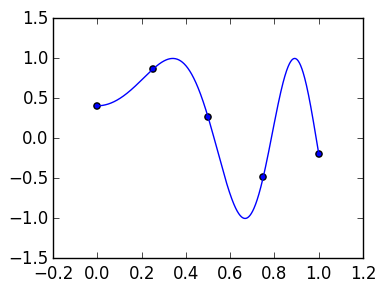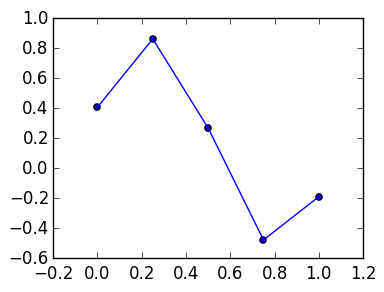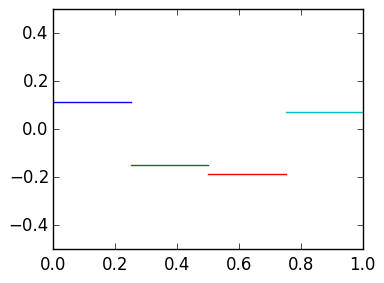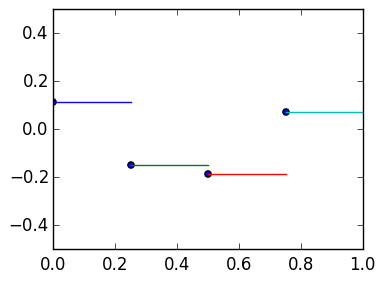# Lecture 34: Discretizing linear ODEs¶

Our approach to solving a linear ODE like

$$u' - a(t) u = f(t), u(0) = c$$

is to replace this infinite-dimensional equation by a finite-dimensional linear system. To do this systematic, we will replicate the procedure of the trapezium rule.

## Trapezium rule revisited¶

The trapezium rule can be thought of as a system of transformations:

1) Discretize: $f(x) \mapsto [f(x_0),\ldots,f(x_n)]^\top$

In :
f=x->cos(20cos(x))
n=4
x=linspace(0.,1.,n+1)
vals=f(x)

g=linspace(0.,1.,1000)

plot(g,f(g))
scatter(x,vals);2) Interpolate: $[f(x_0),\ldots,f(x_n)]^\top \mapsto p(x)$ where $p$ is piecewise affine

In :
scatter(x,vals)
plot(x,vals);3) Integrate: Calculate $\int_0^1 p(x) dx$ exactly

## Discretizing derivatives¶

We want to replicate this procedure to construct an approximation to the derivative of $f(x)$. Note that steps 1 and 2 are the same as before.

1) Discretize: $f(x) \mapsto [f(x_0),\ldots,f(x_n)]^\top$

2) Interpolate: $[f(x_0),\ldots,f(x_n)]^\top \mapsto p(x)$ where $p$ is piecewise affine

3) Differentiate: $p(x) \mapsto p'(x)$ where $p'(x)$ is the exact derivative of $p(x)$

Note that in each panel we have

$$p(x) = f(x_k) + x {f(x_{k+1}) - f(x_k) \over h}\qquad \hbox{for} \qquad x_k \leq x \leq x_{k+1}.$$

Therefore,

$$p'(x) = {f(x_{k+1}) - f(x_k) \over h}\qquad \hbox{for} \qquad x_k \leq x \leq x_{k+1}.$$

Note that the diff(v) command is a convenient shortcut to construct

[v-v,v-v,…,v[end]-v[end-1]]

In :
diff([1,3,7,4])

Out:
3-element Array{Int64,1}:
2
4
-3

Thus the following calculates the values of $p'(x)$ and plots it:

In :
h=1/n

dvals=diff(vals)*h

for k=1:n
plot([(k-1)*h,k*h],[dvals[k],dvals[k]])
end

axis([0,1,-0.5,0.5]);We now add a fourth step: discretize again, but now at $n-1$ points. Because $p'$ jumps at $x_k$, we use the limits from the right, which we denote $x_k+0$.

4) Discretize: $p'(x) \mapsto [p'(x_0 + 0),p'(x_1 + 0),\ldots,p'(x_{n-1}+0)]$

This is precisely the dvals above:

In :
dvals=diff(vals)*h

for k=1:n
plot([(k-1)*h,k*h],[dvals[k],dvals[k]])
end

scatter(x[1:n],dvals)

axis([0,1,-0.5,0.5]);## Discrete derivative as a matrix¶

We can view this as a matrix:

$$D_n \begin{pmatrix} f(x_0) \cr \vdots \cr f(x_n)\end{pmatrix} = \begin{pmatrix} p'(x_0+0) \cr \vdots \cr f(x_{n-1}+0)\end{pmatrix} \approx \begin{pmatrix} f'(x_0) \cr \vdots \cr f'(x_{n-1})\end{pmatrix}$$

for the $n-1 \times n$ matrix

$$D_n \triangleq {1 \over h} \begin{pmatrix} -1 & 1 \cr & -1 & 1 \cr &&\ddots & \ddots \cr &&& -1 & 1\end{pmatrix}$$

## Discrete multiplication¶

We also have to construct a multiplication operator corresponding to multiplication by $a(x)$. Here, the input is a function evaluated at $x_0,\ldots,x_n$ while the output is a function evaluated at $x_0,\ldots,x_{n-1}$, to be consistent with $D_n$. This leads to

$$A_n \begin{pmatrix} f(x_0) \cr \vdots \cr f(x_n)\end{pmatrix} = \begin{pmatrix} a(x_0) f(x_0) \cr \vdots \cr a(x_n) f(x_{n-1})\end{pmatrix}$$

for

$$A_n \triangleq \begin{pmatrix} a_0 \cr & a_1 \cr &&\ddots \cr &&& a_{n-1} & 0\end{pmatrix}$$

where $a_k \triangleq a(x_k)$.

## Approximating the ODE¶

We thus approximate the ODE

$$u'-a(x)u = f(x)$$

to the discrete equation

$$(D_n + A_n) \begin{pmatrix} w_0 \cr \vdots \cr w_n \end{pmatrix} = \begin{pmatrix} f_0 \cr \vdots \cr f_{n-1}\end{pmatrix}$$

where $f_k \triangleq f(x_k)$.

Expanding out the matrix, this gives us

$$\begin{pmatrix} -{1 \over h}-a_0 & {1 \over h} \cr & -{1 \over h}-a_1 & {1 \over h} \cr &&\ddots & \ddots \cr &&& -{1 \over h}-a_{n-1} & {1 \over h}\end{pmatrix}\begin{pmatrix} w_0 \cr \vdots \cr w_n \end{pmatrix} = \begin{pmatrix} f_0 \cr \vdots \cr f_{n-1}\end{pmatrix}$$

This is rectangular, so we need to incorporate the initial condition $u(0) = c$, in other words, we solve the lower triangular system

$$\begin{pmatrix} 1 \cr -{1 \over h}-a_0 & {1 \over h} \cr & -{1 \over h}-a_1 & {1 \over h} \cr &&\ddots & \ddots \cr &&& -{1 \over h}-a_{n-1} & {1 \over h}\end{pmatrix}\begin{pmatrix} w_0 \cr \vdots \cr w_n \end{pmatrix} = \begin{pmatrix} c \cr f_0 \cr \vdots \cr f_{n-1}\end{pmatrix}$$

When $f = 0$ and we multply through by $h$, we see that it is the Euler method.

Next lecture, we will see that replacing the choice of discretization points in step 4 can have dramatic effects.